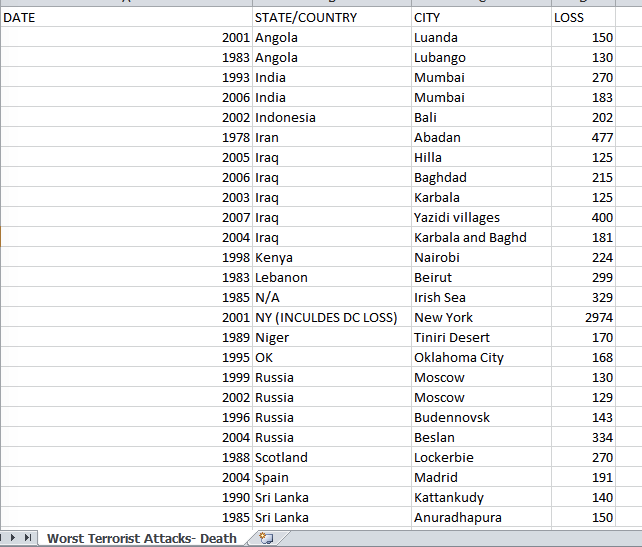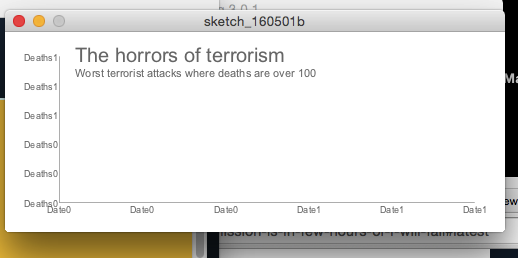#### Howdy, Stranger!

We are about to switch to a new forum software. Until then we have removed the registration on this forum.

# please please can someone help me with this codemy submission is in few hours or i will fail

edited May 2016

I need to make a simple bar chart or anything to put in my sketch every time i try to link the data it gives me errors can you help.

``````PImage img;
Drop[] drops = new Drop;

void setup() {
size(900, 900);
background(0);
stroke(255);
imageMode (CENTER);
for (int i = 0; i < drops.length; i++) {
drops[i] = new Drop();
}
}

void draw() {

background(0);

// Draw the image upper centred of the sketch.
image(img,500,200,1000, 400);
//Change image transparency.
tint(255,127);
for (int i = 0; i < drops.length; i++) {
drops[i].fall();
drops[i].show();
}
}

class Drop {
float x = random(width);
float y = random(-500, -200);
float z = random(0, 20);
float len = map(z, 0, 20, 10, 20);
float yspeed = map(z, 0, 10, 1, 10);

void fall() {
y = y + yspeed;
float grav = map(z, 0, 20, 0, 0.2);
yspeed = yspeed + grav;

if (y > height) {
y = random(-200, -100);
yspeed = map(z, 0, 20, 4, 10);
}
}

void show() {
stroke (127,0,0);
line(x, y, x, y+len);
}
}
``````

I just need any chart like a line graph or anything bar chart to link this datahow can i do this I need to show the Date and the how the deaths increased

Thanks

Tagged:

• every time i try to link the data it gives me errors

there's nothing in the code that links to any data... what have you tried?

and that data is a png - no use to us...

• I didn't include the bar chart code yet I am trying this one but still it doesnt calculate anything. i cant upload a csv file to this fourm :c

import org.gicentre.utils.stat.*; // For chart classes.

// Displays a simple line chart representing a time series.

XYChart lineChart;

// Loads data into the chart and customises its appearance. void setup() { size(500,200); textFont(createFont("Arial",10),10);

// Both x and y data set here.
lineChart = new XYChart(this); lineChart.setData(new float[] {1970, 1980, 1990, 2000, 2002, 2003, 2004, 2005, 2006, 2007}, new float[] { 100, 200, 300, 400, 500, 600, 700, 800, 900, 1000, 2000});

// Axis formatting and labels. lineChart.showXAxis(true); lineChart.showYAxis(true); lineChart.setMinY(0);

lineChart.setYFormat("Deaths"); // Monetary value in \$US lineChart.setXFormat("Date"); // Year

// Symbol colours lineChart.setPointColour(color(180,50,50,100)); lineChart.setPointSize(5); lineChart.setLineWidth(2); }

// Draws the chart and a title. void draw() { background(255); textSize(9); lineChart.draw(15,15,width-30,height-30);

// Draw a title over the top of the chart. fill(120); textSize(20); text("The horrors of terrorism", 70,30); textSize(11); text("Worst terrorist attacks where deaths are over 100", 70,45); }

• just post the 5 lines of the csv?

• I am the number one beginner in this sorry guys i know this is stupid, this is the code i'm working on in the image it doesnt write the years and the number of deaths how do i fix that? and how do I actually write the function to present the line chart

import org.gicentre.utils.stat.*; // For chart classes.

// Displays a simple line chart representing a time series.

XYChart lineChart;

// Loads data into the chart and customises its appearance. void setup() { size(500,200); textFont(createFont("Arial",10),10);

// Both x and y data set here.
lineChart = new XYChart(this); lineChart.setData(new float[] {1970, 1980, 1990, 2000, 2002, 2003, 2004, 2005, 2006, 2007}, new float[] { 100, 200, 300, 400, 500, 600, 700, 800, 900, 1000, 2000});

// Axis formatting and labels. lineChart.showXAxis(true); lineChart.showYAxis(true); lineChart.setMinY(0);

lineChart.setYFormat("Deaths"); // Monetary value in \$US lineChart.setXFormat("Date"); // Year

// Symbol colours lineChart.setPointColour(color(180,50,50,100)); lineChart.setPointSize(5); lineChart.setLineWidth(2); }

// Draws the chart and a title. void draw() { background(255); textSize(9); lineChart.draw(15,15,width-30,height-30);

// Draw a title over the top of the chart. fill(120); textSize(20); text("The horrors of terrorism", 70,30); textSize(11); text("Worst terrorist attacks where deaths are over 100", 70,45); }